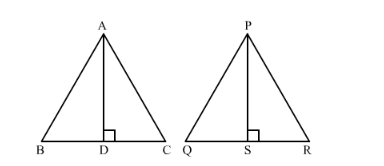# Two isosceles triangles have equal angles and their areas are in the ratio 16 : 25. The ratio of their corresponding heights is

Question:

Two isosceles triangles have equal angles and their areas are in the ratio 16 : 25. The ratio of their corresponding heights is

(a) 4 : 5
(b) 5 : 4
(c) 3 : 2
(d) 5 : 7

Solution:

Given: Two isosceles triangles have equal vertical angles and their areas are in the ratio of 16:25.

To find: Ratio of their corresponding heights.Let ∆ABC and ∆PQR be two isosceles triangles such that ∠A=∠P. Suppose AD ⊥ BC and PS ⊥ QR .

In ∆ABC and ∆PQR,

ABPQ=ACPR∠A=∠P∴∆ABC~∆PQR       SAS similarity

We know that the ratio of areas of two similar triangles is equal to the ratio of squares of their corresponding altitudes.

Hence,

Hence we got the result as $(a)$